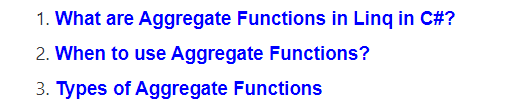# LINQ Aggregate Functions in C#

## LINQ Aggregate Functions in C#

In this article, I am going to discuss the LINQ Aggregate Functions in C# with Examples. Please read our previous article before proceeding to this article where we discussed the LINQ Reverse Method in C# with Examples. As part of this article, we are going to discuss the following concepts.##### What are LINQ Aggregate Functions in C#?

The LINQ Aggregate Functions are used to group together the values of multiple rows as the input and then return the output as a single value. So, in simple words, we can say that the aggregate function in C# is always going to return a single value.

##### When to use the Aggregate Functions in C#?

Whenever you want to perform some mathematical operations such as Sum, Count, Max, Min, Average, and Aggregate on the numeric property of a collection then you need to use the Linq Aggregate Functions.

##### What are the Aggregate Methods Provided by Linq?

The following are the aggregate methods provided by Linq to perform mathematical operations on a collection.

1. Sum(): This method is used to calculate the total(sum) value of the collection.
2. Max(): This method is used to find the largest value in the collection
3. Min(): This method is used to find the smallest value in the collection
4. Average(): This method is used to calculate the average value of the numeric type of the collection.
5. Count(): This method is used to count the number of elements present in the collection.
6. Aggregate(): This method is used to Perform a custom aggregation operation on the values of a collection.

In the next article, I am going to discuss the Linq Sum Aggregate Method in detail.